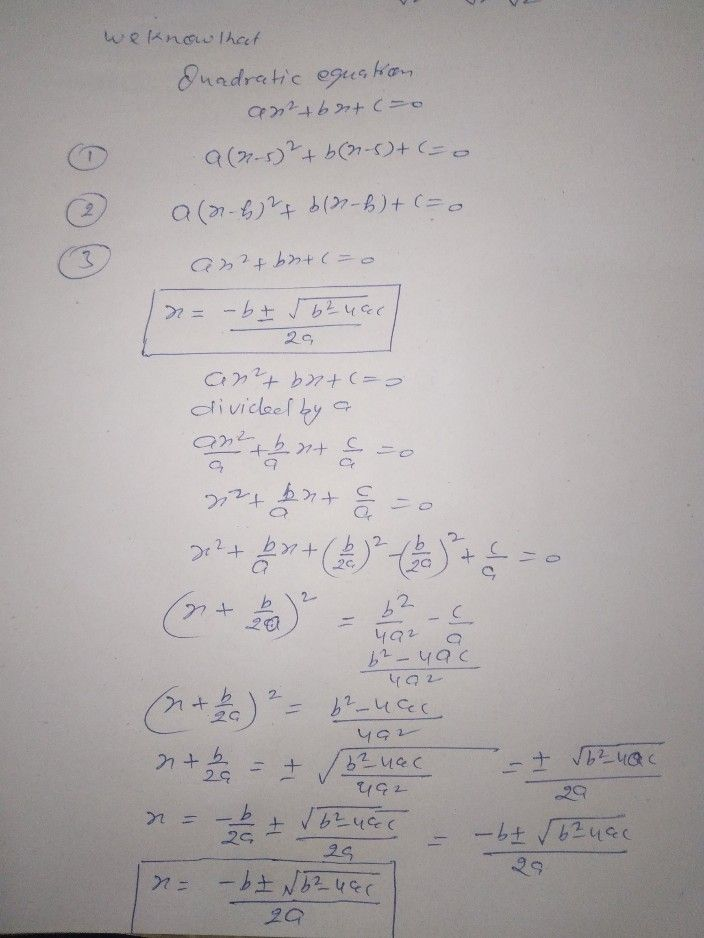Symbol
Problem$tcts$ Do the $Prob1em$ $0$ $1$ $e2k0$ situation where the concept of a rational algebraic equation $0n$ $10\pi$ $ne10$ a quadratic equation is being applied. $s0$ the situation to answer $90os60ns$ 1. How is the concept of a rational algebraic equation $\pi ns40$ $=$ in quadratic equation applied in the situation? $2$ What quantities are invoived in the situation? Which of these quantitis e $00\pi 3$ How about the quantities that are $10$ $n0$ $\pi$ $2$ Formulate then solve the problem out of the given. $4$ What do the solution obtained represent? Explain your answer. $Ra6nc$ $Pca1-11$ situation involving rational algebraic equations $ans10$ into quadratic equations.
7th-9th grade
Algebra
Search count: 136
SolutionQanda teacher - LokeshStudent
thank you